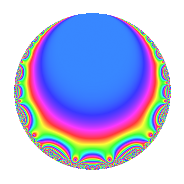# Properties

 Label 349.2.b.aLevel 349 Weight 2 Character orbit 349.b Analytic conductor 2.787 Analytic rank 0 Dimension 2 CM No Inner twists 2

# Related objects

## Newspace parameters

 Level: $$N$$ = $$349$$ Weight: $$k$$ = $$2$$ Character orbit: $$[\chi]$$ = 349.b (of order $$2$$ and degree $$1$$)

## Newform invariants

 Self dual: No Analytic conductor: $$2.78677903054$$ Analytic rank: $$0$$ Dimension: $$2$$ Coefficient field: $$\Q(\sqrt{-5})$$ Coefficient ring: $$\Z[a_1, \ldots, a_{7}]$$ Coefficient ring index: $$2$$ Sato-Tate group: $\mathrm{SU}(2)[C_{2}]$

## $q$-expansion

Coefficients of the $$q$$-expansion are expressed in terms of $$\beta = 2\sqrt{-5}$$. We also show the integral $$q$$-expansion of the trace form.

 $$f(q)$$ $$=$$ $$q - q^{3} + 2 q^{4} + 2 q^{5} + \beta q^{7} -2 q^{9} +O(q^{10})$$ $$q - q^{3} + 2 q^{4} + 2 q^{5} + \beta q^{7} -2 q^{9} + \beta q^{11} -2 q^{12} -\beta q^{13} -2 q^{15} + 4 q^{16} + 3 q^{17} -5 q^{19} + 4 q^{20} -\beta q^{21} + q^{23} - q^{25} + 5 q^{27} + 2 \beta q^{28} + q^{29} + 7 q^{31} -\beta q^{33} + 2 \beta q^{35} -4 q^{36} -3 q^{37} + \beta q^{39} + 10 q^{41} -\beta q^{43} + 2 \beta q^{44} -4 q^{45} -2 \beta q^{47} -4 q^{48} -13 q^{49} -3 q^{51} -2 \beta q^{52} -\beta q^{53} + 2 \beta q^{55} + 5 q^{57} + \beta q^{59} -4 q^{60} -2 \beta q^{61} -2 \beta q^{63} + 8 q^{64} -2 \beta q^{65} -13 q^{67} + 6 q^{68} - q^{69} -2 \beta q^{71} -11 q^{73} + q^{75} -10 q^{76} -20 q^{77} -\beta q^{79} + 8 q^{80} + q^{81} + q^{83} -2 \beta q^{84} + 6 q^{85} - q^{87} + 2 \beta q^{89} + 20 q^{91} + 2 q^{92} -7 q^{93} -10 q^{95} -3 \beta q^{97} -2 \beta q^{99} +O(q^{100})$$ $$\operatorname{Tr}(f)(q)$$ $$=$$ $$2q - 2q^{3} + 4q^{4} + 4q^{5} - 4q^{9} + O(q^{10})$$ $$2q - 2q^{3} + 4q^{4} + 4q^{5} - 4q^{9} - 4q^{12} - 4q^{15} + 8q^{16} + 6q^{17} - 10q^{19} + 8q^{20} + 2q^{23} - 2q^{25} + 10q^{27} + 2q^{29} + 14q^{31} - 8q^{36} - 6q^{37} + 20q^{41} - 8q^{45} - 8q^{48} - 26q^{49} - 6q^{51} + 10q^{57} - 8q^{60} + 16q^{64} - 26q^{67} + 12q^{68} - 2q^{69} - 22q^{73} + 2q^{75} - 20q^{76} - 40q^{77} + 16q^{80} + 2q^{81} + 2q^{83} + 12q^{85} - 2q^{87} + 40q^{91} + 4q^{92} - 14q^{93} - 20q^{95} + O(q^{100})$$

## Character Values

We give the values of $$\chi$$ on generators for $$\left(\mathbb{Z}/349\mathbb{Z}\right)^\times$$.

 $$n$$ $$2$$ $$\chi(n)$$ $$-1$$

## Embeddings

For each embedding $$\iota_m$$ of the coefficient field, the values $$\iota_m(a_n)$$ are shown below.

For more information on an embedded modular form you can click on its label.

Label $$\iota_m(\nu)$$ $$a_{2}$$ $$a_{3}$$ $$a_{4}$$ $$a_{5}$$ $$a_{6}$$ $$a_{7}$$ $$a_{8}$$ $$a_{9}$$ $$a_{10}$$
348.1
 − 2.23607i 2.23607i
0 −1.00000 2.00000 2.00000 0 4.47214i 0 −2.00000 0
348.2 0 −1.00000 2.00000 2.00000 0 4.47214i 0 −2.00000 0
 $$n$$: e.g. 2-40 or 990-1000 Significant digits: Format: Complex embeddings Normalized embeddings Satake parameters Satake angles

## Inner twists

Char. orbit Parity Mult. Self Twist Proved
1.a Even 1 trivial yes
349.b Even 1 yes

## Hecke kernels

This newform can be constructed as the kernel of the linear operator $$T_{2}$$ acting on $$S_{2}^{\mathrm{new}}(349, [\chi])$$.